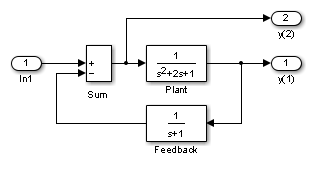The Simulink® `trim` function uses a model to determine steady-state points of a dynamic system that satisfy input, output, and state conditions that you specify. Consider, for example, this model, called `ex_lmod`.You can use the `trim` function to find the values of the input and the states that set both outputs to 1. First, make initial guesses for the state variables (`x`) and input values (`u`), then set the desired value for the output (`y`).

```x = [0; 0; 0]; u = 0; y = [1; 1]; ```

Use index variables to indicate which variables are fixed and which can vary.

```ix = []; % Don't fix any of the states iu = []; % Don't fix the input iy = [1;2]; % Fix both output 1 and output 2 ```

Invoking `trim` returns the solution. Your results might differ because of roundoff error.

```[x,u,y,dx] = trim('lmod',x,u,y,ix,iu,iy) x = 0.0000 1.0000 1.0000 u = 2 y = 1.0000 1.0000 dx = 1.0e-015 * -0.2220 -0.0227 0.3331 ```

Note that there might be no solution to equilibrium point problems. If that is the case, `trim` returns a solution that minimizes the maximum deviation from the desired result after first trying to set the derivatives to zero. For a description of the `trim` syntax, see `trim`.